# Electronics and Communication Engineering - Microwave Communication

### Exercise :: Microwave Communication - Section 3

11.

A coaxial RF cable has a characteristic impedance of 50 Ω and C equal to 40 pF/m. The inductance is

 A. 1 μ H/m B. 10 μ H/m C. 0.1 μ H/m D. 0.01 μ H/m

Explanation: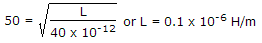.

12.

In a microwave coaxial line, the maximum operating frequency is f max and breakdown strength of dielectric is Ed' Then maximum power handling capacity is proportional to

 A.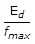B.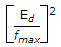C.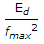D.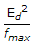Explanation:

Power handling capacity μ (voltage)2 and is inversely proportional to f2 max.

13.

To couple a coaxial line to a parallel wire line it is best to use

 A. slotted line B. balun C. directional coupler D. λ/4 transformer

Explanation:

A balun gives 4 : 1 impedance transformation.

14.

In a multicavity klystron amplifier the signal to be amplified develops an ac voltage of signal frequency across the gap in buncher cavity

 A. True B. False

Explanation:

A Klystron is a vacuum tube used for generation/amplification of microwaves.

An electron beam is produced by oxide coated indirectly heated cathode and is focussed and accelerated by focussing electrode.

This beam is transmitted through a glass tube. The input cavity where the beam enters the glass tube is called buncher.

As electrons move ahead they see an accelerating field for half cycle and retarding field for the other half cycle.

Therefore, some electrons are accelerated and some are retarded. This process is called velocity modulation.

The velocity modulation causes bunching of electrons. This bunching effect converts velocity modulation into density modulation of beam.

The input is fed at buncher cavity and output is taken at catcher cavity.

In a two cavity klystron only buncher and catcher cavity are used. In multi cavity klystron one or more intermediate cavities are also used.

The features of a multicavity klystron are :

1. Frequency range - 0.25 GHz to 100 GHz

2. Power output - 10 kW to several hundred kW

3. Power gain - 60 dB (nominal value)

A multicavity klystron is used in UHF TV transmitters, Radar transmitter and satellite communication.

15.

If VSWR is infinite, the transmission line is terminated in

 A. short circuit B. complex impedance C. open circuit D. either (a) or (c)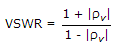and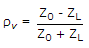. Since VSWR = ∞, |rv| = 1. This can happen if ZL = 0 or ZL = ∞, i.e., line is o.c or s.c.